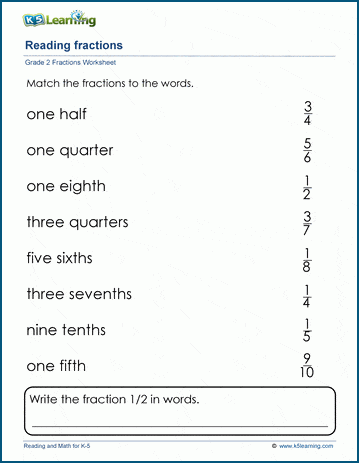## Matching fractions to their words

In these grade 2 math worksheets students match fractions in standard form (numerator and denominator) with fractions written out in words.  Students learn that "1/2" is the same as "one half" and so on.  Reading fractions fluently in both forms is a basic skill needed for further study of fractions.## More fractions worksheets

Explore all of our fractions worksheets, from dividing shapes into "equal parts" to multiplying and dividing improper fractions and mixed numbers.

## What is K5?

K5 Learning offers reading and math worksheets, workbooks and an online reading and math program for kids in kindergarten to grade 5.  We help your children build good study habits and excel in school.